(diff) ← Older revision | Latest revision (diff) | Newer revision → (diff)

## Overview

A fast Fourier transform (FFT) is a method to calculate a discrete Fourier transform (DFT). More information about FFTs and DFTs can be found on wikipedia (linked). The following circuit and code allow a user to put a signal into a PIC32, perform an FFT on that signal, output the data to Matlab via RS-232, and view a plot showing the raw signal, the FFT as calculated by the PIC, and the FFT as calculated by Matlab. Viewing the Matlab calculation is for verification only and can be commented out of the Matlab code.

Commented C code and Matlab code for a FFT are provided in the FFT Code section of this page and can be downloaded here.

With the PIC32, an inverse FFT can also be computed. Inverse FFT is a function that converts a frequency domain signal into a time domain signal. Inverse FFTs actually use the same algorithm as FFTs except with some scaling and conjugation or time reversal (depending on the algorithm). The second half of this page shows how you can create an analog signal, takes the FFT of that signal, and then take the inverse FFT of the result to try and obtain the original waveform back. The data is all sent to MATLAB via RS232 and compared to MATLAB's computational results for accuracy.

The C code and Matlab code for this program can be downloaded here.

## FFT Circuit

Beyond simple inputs and outputs, there is no special circuitry required for computing FFTs. The signal is inserted into pin B4 on the PIC and must be below VREF on the PIC (currently configured to 3.3 V). For data output, the PIC uses RS-232 communication. Three connections need to be made with the RS-232:

• RS-232 Tx to pin F4 on the PIC (orange connection in diagram)
• RS-232 Rx to pin F5 on the PIC (yellow connection in diagram)
• Black RS-232 wire to ground

The remaining connection is not essential for FFT caclulation but allows the user to monitor the calculation time. Pin A14 goes high during calculation and goes back low when the calculation is completed. This feature lets the calculation time be monitored on an oscilloscope.

The following circuit diagram illustrates the signal generator to PIC connection, the RS-232 connections, and the optional oscilloscope connection.

## FFT Code

The following is the source code to be programmed onto the PIC. If unfamiliar, see here for a guide to creating a .hex file with MPLAB and here for directions to load files to the PIC. It first establishes inputs, outputs and RS-232 communication. When prompted with a 'p' (as written, it is prompted automatically by Matlab), the PIC computes the FFT then sends the magnitude portion of the single-sided FFT and the raw samples to Matlab.

```/* Sam Bobb,  Daniel Cornew,  Ryan Deeter
Fast Fourier Transform (FFT) Example
ME 333, Lab 5
Feb 2010
*/

/** INCLUDES ***************************************************/
#include "HardwareProfile.h"
#include <plib.h>
#include "dsplib_dsp.h"
#include "fftc.h"

/** Constants **************************************************/

#define TRUE 		1
#define FALSE		0

#define LOOP_TIME_PIN		LATAbits.LATA14

#define DESIRED_BAUDRATE    	(19200)      // The desired BaudRate

// To modify the number of samples:
#define fftc fft16c256 //from fftc.h, for N = 256 use fft16c256, for N = 1024 use fft16c1024
#define N 256	// Also change the log2N variable below!!

// To modify the sampling frequency
#define SAMPLE_FREQ 10000
// Also change the timer interupt setup in initInterruptController!

/** Function Declarations **************************************/
void initInterruptController();

void initUART2(int pbClk);

void sendDataRS232(void);

void computeFFT(void);  //sets up function to compute FFT

/** Global Variables *******************************************/

int computeFFTflag = FALSE; //used to indicate that an fft computation has been requested over rs232

int sampleIndex = 0; //keeps track of where we're putting our ADC reading

/** establish variables for FFT calculation*/
// -----
// this has to be changed for different values of N
int log2N = 8; // log2(256) = 8
//int log2N = 10; // log2(1024) = 10
// -----

int16c sampleBuffer[N]; //initialize buffer to collect samples
int16c calcBuffer[N]; // initialize buffer to hold old samples
int16c scratch[N];
int16c dout[N]; //holds computed FFT until transmission

long int singleSidedFFT[N];
long int freqVector[N];

/** Main Function **********************************************/

int main(void)
{
int	pbClk;
int i;

// Configure the proper PB frequency and the number of wait states
pbClk = SYSTEMConfigPerformance(SYS_FREQ);

TRISAbits.TRISA14 = 0;

// Allow vector interrupts
INTEnableSystemMultiVectoredInt();

mInitAllLEDs();

initInterruptController();

initUART2(pbClk);

// Configure the proper PB frequency and the number of wait states
SYSTEMConfigPerformance(SYS_FREQ);

// -------- Set Up ADC --------
// configure and enable the ADC

// define setup parameters for OpenADC10
// Turn module on | output in integer | trigger mode auto | enable  autosample

// define setup parameters for OpenADC10
// ADC ref external    | disable offset test    | enable scan mode | perform 2 samples | use one buffer | use MUXA mode

// define setup parameters for OpenADC10
// 				  use ADC internal clock | set sample time

// define setup parameters for OpenADC10
// set AN4
#define PARAM4	ENABLE_AN4_ANA

// define setup parameters for OpenADC10
// do not assign channels to scan
#define PARAM5	SKIP_SCAN_AN0 | SKIP_SCAN_AN1 | SKIP_SCAN_AN2 | SKIP_SCAN_AN3 | SKIP_SCAN_AN5 | SKIP_SCAN_AN6 | SKIP_SCAN_AN7 | SKIP_SCAN_AN8 | SKIP_SCAN_AN9 | SKIP_SCAN_AN10 | SKIP_SCAN_AN11 | SKIP_SCAN_AN12 | SKIP_SCAN_AN13 | SKIP_SCAN_AN14 | SKIP_SCAN_AN15

// use ground as neg ref for A | use AN4 (B4) for input A
// configure to sample AN4
OpenADC10( PARAM1, PARAM2, PARAM3, PARAM4, PARAM5 ); // configure ADC using parameter define above

while ( ! mAD1GetIntFlag() ) { } // wait for the first conversion to complete so there will be valid data in ADC result registers
// ------ DONE SETTING UP ADC ------

// zero the freqVector and singleSidedFFT
for (i=0; i<N; i++)
{
freqVector[i] = 0;
singleSidedFFT[i] = 0;
}

// generate frequency vector
// this is the x-axis of your single sided fft
for (i=0; i<N/2; i++)
{
freqVector[i] = i*(SAMPLE_FREQ/2)/((N/2) - 1);
}

// wait around until you get an fft command from rs232
// then call computeFFT to generate an fft of the last block of samples
// then send out rs232.
// If you want to use FFT without rs232, you can call computeFFT when ever you want in your program.
while(1)
{
if (computeFFTflag == TRUE)
{
computeFFT();
sendDataRS232();
}
}

CloseOC1();

} //end main

/** Interrupt Handlers *****************************************/
// interrput code for the timer 3
void __ISR( _TIMER_3_VECTOR, ipl7) T3Interrupt( void)
{
int i;
//LOOP_TIME_PIN = TRUE;
// When we used the loop time pin to measure the length of this ISR,
// we measured 400 ns, so you could sample at over 1 MHz.

sampleBuffer[sampleIndex].im = 0; // the imaginary value is 0.

// you could shave a little time off this ISR by just zeroing the .im value once, outside the ISR

// increment the sampleIndex
if (sampleIndex == (N-1))
{
sampleIndex = 0;
}
else
{
sampleIndex++;
}

//LOOP_TIME_PIN = FALSE;

// clear interrupt flag and exit
mT3ClearIntFlag();
} // T3 Interrupt

// UART 2 interrupt handler
// it is set at priority level 2
void __ISR(_UART2_VECTOR, ipl2) IntUart2Handler(void)
{
char data;

// Is this an RX interrupt?
if(mU2RXGetIntFlag())
{
// Clear the RX interrupt Flag
mU2RXClearIntFlag();

// Echo what we just received.
putcUART2(data);

switch(data)
{

case 'p': // compute and output the FFT
computeFFTflag = TRUE;
break;
}

// Toggle LED to indicate UART activity
mLED_0_Toggle();

}

// We don't care about TX interrupt
if ( mU2TXGetIntFlag() )
{
mU2TXClearIntFlag();
}
}

/** Other Functions ********************************************/

void initInterruptController(void)
{
// init Timer3 mode and period (PR3)
OpenTimer3( T3_ON | T3_PS_1_1 | T3_SOURCE_INT, 0x1F40); // produces 100 ms period
// sampling frequency = 10 kHz

mT3SetIntPriority( 7); 	// set Timer3 Interrupt Priority
mT3ClearIntFlag(); 		// clear interrupt flag
mT3IntEnable( 1);		// enable timer3 interrupts
}

void initUART2(int pbClk)
{
// define setup Configuration 1 for OpenUARTx
// Module Enable
// Work in IDLE mode
// Communication through usual pins
// Disable wake-up
// Loop back disabled
// Input to Capture module from ICx pin
// no parity 8 bit
// 1 stop bit
// IRDA encoder and decoder disabled
// CTS and RTS pins are disabled
// UxRX idle state is '1'
// 16x baud clock - normal speed
#define config1 	UART_EN | UART_IDLE_CON | UART_RX_TX | UART_DIS_WAKE | UART_DIS_LOOPBACK | UART_DIS_ABAUD | UART_NO_PAR_8BIT | UART_1STOPBIT | UART_IRDA_DIS | UART_DIS_BCLK_CTS_RTS| UART_NORMAL_RX | UART_BRGH_SIXTEEN

// define setup Configuration 2 for OpenUARTx
// IrDA encoded UxTX idle state is '0'
// Enable UxRX pin
// Enable UxTX pin
// Interrupt on transfer of every character to TSR
// Interrupt on every char received
// Rx Buffer Over run status bit clear
#define config2		UART_TX_PIN_LOW | UART_RX_ENABLE | UART_TX_ENABLE | UART_INT_TX | UART_INT_RX_CHAR | UART_ADR_DETECT_DIS | UART_RX_OVERRUN_CLEAR

// Open UART2 with config1 and config2
OpenUART2( config1, config2, pbClk/16/DESIRED_BAUDRATE-1);	// calculate actual BAUD generate value.

// Configure UART2 RX Interrupt with priority 2
ConfigIntUART2(UART_INT_PR2 | UART_RX_INT_EN);
}

void sendDataRS232()
{
int i;
char RS232_Out_Buffer; // max characters per line (line feed and carriage return count)

sprintf(RS232_Out_Buffer,"\n\rSTART\n\r"); //print START. MATLAB uses this as a start delimiter
putsUART2(RS232_Out_Buffer);

sprintf(RS232_Out_Buffer,"ROWS=%d\n\r", N); //print the number of rows, so matlab can make the correct sized buffer
putsUART2(RS232_Out_Buffer);

for(i = 0; i < N; i++)
{
// output: frequency vector, the calculated fft, raw samples
sprintf(RS232_Out_Buffer,"%d %d %d\n\r",freqVector[i], singleSidedFFT[i], calcBuffer[i].re);
putsUART2(RS232_Out_Buffer);
}

sprintf(RS232_Out_Buffer,"END\n\r"); //output end so matlab knows we're done
putsUART2(RS232_Out_Buffer);

}

void computeFFT()
{
// when using 256 samples, we measured this function to take about 500 microseconds
// (not including the time to send rs232 data)
int i;

mT3IntEnable(0); //turns off interrupt while computing FFT

LOOP_TIME_PIN = TRUE;

for (i=0; i<N; i++)
{
if (i<sampleIndex)
{
// old chunk
calcBuffer[i+(N-sampleIndex)] = sampleBuffer[i];
}
else // i >= sampleIndex
{
// new chunk
calcBuffer[i-sampleIndex] = sampleBuffer[i];
}

}

// load complex input data into din
mips_fft16(dout, calcBuffer, fftc, scratch, log2N);

// compute single sided fft
for(i = 0; i < N/2; i++)
{
singleSidedFFT[i] = 2 * ((dout[i].re*dout[i].re) + (dout[i].im*dout[i].im));
}

LOOP_TIME_PIN = FALSE;

computeFFTflag = FALSE;

// do something with dout
mT3IntEnable(1); //turn interrupt back on
}

```

The following Matlab code computes its own FFT with the raw samples. It then plots the input signal, the PIC calculated FFT, and the Matlab calculated FFT, for comparison.

```
% fftserial interface v 2

%Sam Bobb, Daniel Cornew, Ryan Deeter

%This code receives the single sided magnitude FFT calculated on the PIC
%and the raw samples through serial communication.  Matlab calculates its
%own FFT of the raw samples, then plots the input signal, PIC calculated
%FFT, and Matlab calculated FFT.

Fs = 10000;                    % Sampling frequency
T = 1/Fs;                     % Sample time
L = 256;                     % Length of signal
t = (0:L-1)*T;                % Time vector

%for runtimes=1:100      %UNCOMMENT FOR CONTINUOUS FFT

columns = 3;

%check if the serial port already exists
%if it does, close it, it probably means something bad happened last run
if exist('COM','var')
disp('closing old port instance');
fclose(COM)
delete(COM)
clear COM
disp('port closed');
end

% open the serial port
COM = serial('COM6');
set(COM,'BaudRate',19200);
fopen(COM)

disp('port opened');

% send the command to print data
fprintf(COM,'p')

%there's usually some garbage at the beginning (like the echoed p
%character command and line  breaks); this reads lines in until we find
%the start line
text = fscanf(COM, '%s');
while isempty(strfind(text, 'START'))
text = fscanf(COM, '%s');
end

rowstext = fscanf(COM, '%s');
pat = 'ROWS=';
split = regexp(rowstext, pat, 'split');
rows = str2double(split(2));

DATA = zeros(rows,columns);

%generate the fscanf format argument
%repeate the formatchar character for the number of columns
formatchar = '%d';
serialpat = '';

%establishing fscanf format
for j = 1:columns
serialpat = [serialpat ' ' formatchar];
end

%reads serial data and puts it into DATA
for j = 1:rows
DATA(j, :) = fscanf(COM, serialpat, [columns 1]);
%disp(COM.BytesAvailable)

end

last = fscanf(COM, '%s');

%verifies that last line read is the last line
if strcmp(last,'END')
disp('Success')
else
disp('Problem')
end

%disp(COM.BytesAvailable)       %for debugging

%plotting input signal
subplot(3, 1, 1)
plot(DATA(:,3))
title('Time Domain Signal')

%plotting PIC calculated FFT
subplot(3, 1, 2)
plot(DATA(:,1), DATA(:,2))
title('Single Sided FFT Calculated on the PIC')
xlabel('Frequency (Hz)')
ylabel('|Y(f)|')
axis([0 Fs/2 0 2e5])

%calculating FFT in Matlab
NFFT = 2^nextpow2(L); % Next power of 2 from length of y
Y = fft(DATA(:,3),NFFT)/L;

%Y = DATA(:,1) + DATA(:,2)*1i;

f = Fs/2*linspace(0,1,NFFT/2+1);

% Plot Matlab's single-sided amplitude spectrum.
subplot(3, 1, 3)
plot(f,2*abs(Y(1:NFFT/2+1)))
title('Single Sided FFT Calculated with MATLAB')
xlabel('Frequency (Hz)')
ylabel('|Y(f)|')

%pause(2)        %UNCOMMENT FOR CONTINOUS FFT

%end             %UNCOMMENT FOR CONTINUOUS FFT

%closes serial port
fclose(COM)
delete(COM)
clear COM
disp('port closed');

```

## FFT Example Plots

Here is an example using a 500 Hz sine wave input.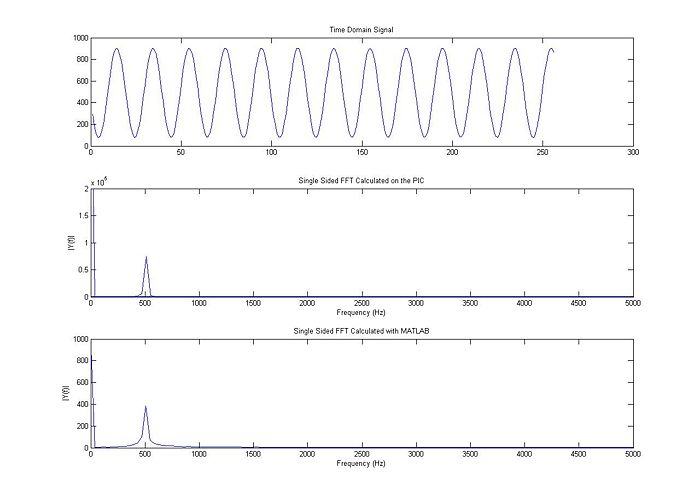This example uses a 1 kHz sine wave.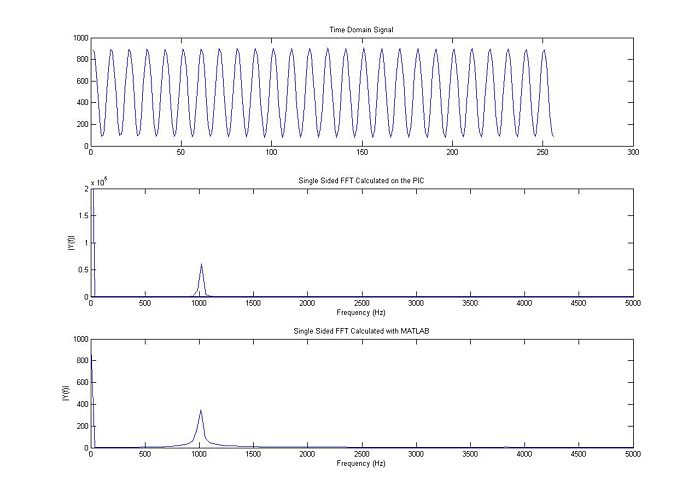This example is still at 1 kHz, but with a square wave.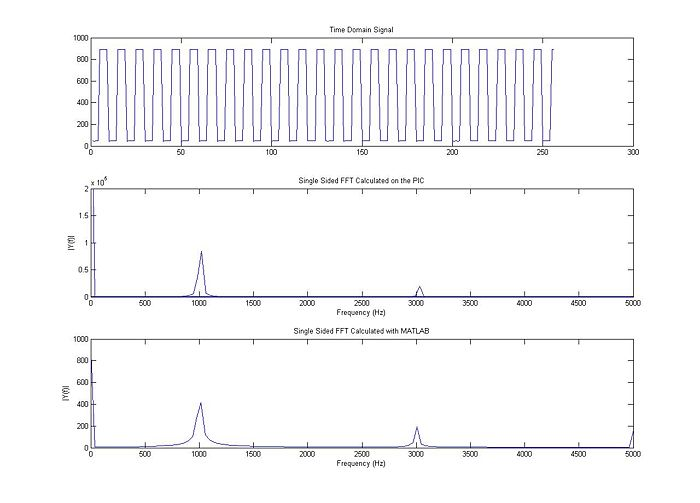This example shows a 2 kHz sine wave.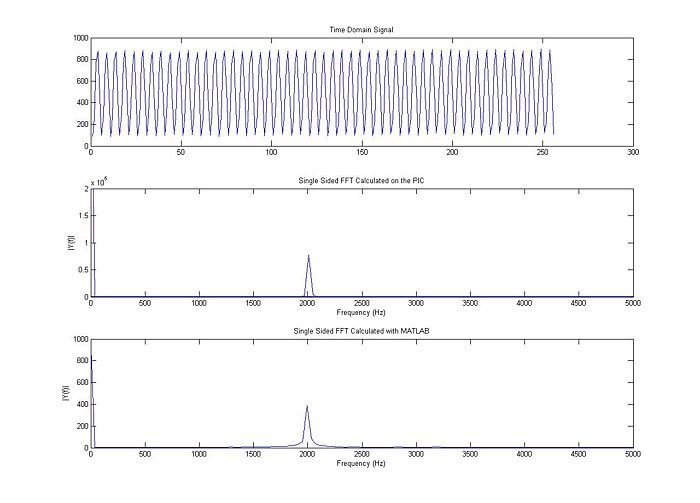This example, still at 2 kHz, is a square wave.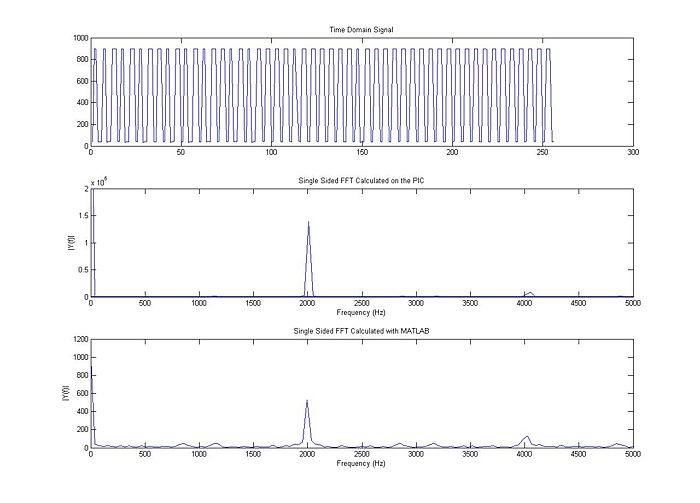The final example is a 3 kHz sine wave.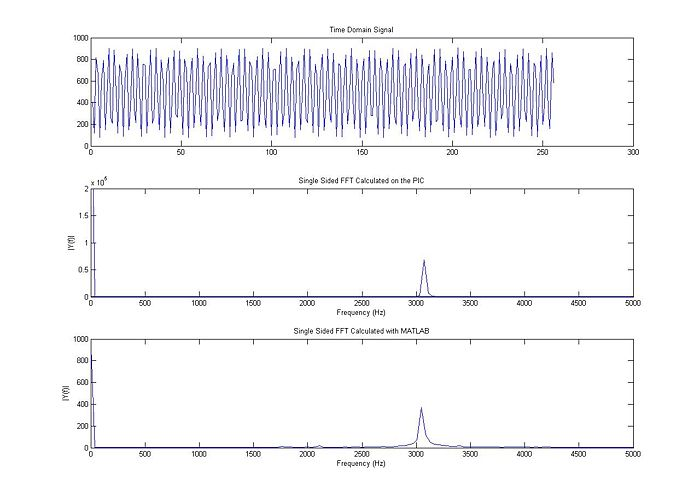## IFFT Circuit

This circuit is very similar to the preview FFT circuit, except that it includes a low pass filter. This filter integrates the PWM signal that is output from pin D0 and creates a continuous, analog signal.

## IFFT Code

The code for both MATLAB and the PIC32 can be found here. The code is very similar to the code found above. There are two main differences:

1. There is now an "analog output." This is basically a PWM that is filtered through a low pass filter. The pulse widths are modified very quickly, with the width of the pulse directly related to the amplitude of the output. Thus, a very short pulse is the same as the trough of the sine wave and a long pulse is the same as the peak of the wave.

2. This is more of a tip, but after the analog inputs are stored in the sample array, they are scaled up by a factor, in this case, 256. All this does is increase the range of the values. I found this to give results that align much better with MATLAB's results. The reason for this is that the mips_fft16 algorithm accepts int16 and int32 data the best. However, int data types can lose information since they round to the nearest integer. Thus, we can scale all the inputs to make them larger, thus yielding more accuracy (it is as if we added a few more decimal places).

## IFFT Example Plot

This waveform is a 30Hz sine wave superimposed on a 40Hz sine wave with the same amplitude and phase. As one can see, the FFT amplitude line up almost exactly. The phase graph has some discrepancy, but this can be easily fixed by changing the FFT phase vector to float, rather than int16. It is ok to use int16 here because the vector is not being inputted into the mips_fft algorithm. And finally, the inverse FFT matches up very nicely with the original signal, very little information is lost.